SSC CGL Previous Year Questions: Average- 1

# SSC CGL Previous Year Questions: Average- 1 - SSC CGL

Test Description

## 20 Questions MCQ Test SSC CGL (Tier - 1) - Previous Year Papers (Topic Wise) - SSC CGL Previous Year Questions: Average- 1

SSC CGL Previous Year Questions: Average- 1 for SSC CGL 2023 is part of SSC CGL (Tier - 1) - Previous Year Papers (Topic Wise) preparation. The SSC CGL Previous Year Questions: Average- 1 questions and answers have been prepared according to the SSC CGL exam syllabus.The SSC CGL Previous Year Questions: Average- 1 MCQs are made for SSC CGL 2023 Exam. Find important definitions, questions, notes, meanings, examples, exercises, MCQs and online tests for SSC CGL Previous Year Questions: Average- 1 below.
Solutions of SSC CGL Previous Year Questions: Average- 1 questions in English are available as part of our SSC CGL (Tier - 1) - Previous Year Papers (Topic Wise) for SSC CGL & SSC CGL Previous Year Questions: Average- 1 solutions in Hindi for SSC CGL (Tier - 1) - Previous Year Papers (Topic Wise) course. Download more important topics, notes, lectures and mock test series for SSC CGL Exam by signing up for free. Attempt SSC CGL Previous Year Questions: Average- 1 | 20 questions in 20 minutes | Mock test for SSC CGL preparation | Free important questions MCQ to study SSC CGL (Tier - 1) - Previous Year Papers (Topic Wise) for SSC CGL Exam | Download free PDF with solutions
 1 Crore+ students have signed up on EduRev. Have you?
SSC CGL Previous Year Questions: Average- 1 - Question 1

### The average of four numbers is 20. If the average of the first two numbers is 15, then what is the average of the last two numbers?        (SSC MTS 2018)

Detailed Solution for SSC CGL Previous Year Questions: Average- 1 - Question 1

Let the 4 no's be x1, x2, x3 and x4
Average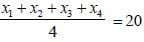x1 + x2 + x3 + x4 = 80
Average of first two numbers are 15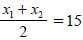x1 + x2 = 30 ...(1)
x1 + x2 + x3 + x4 = 80
From the eq. (1) we have put the value of
x1 + x2 = 30
x3 + x4 = 80 – 30
x3 + x4 = 50
Hence the average of last two no. is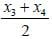= 50/2 = 25

SSC CGL Previous Year Questions: Average- 1 - Question 2

### The ratio of the age of a father and his son is 3 : 1. If the product of their ages is 432, then what is the sum of their ages?                     (SSC MTS 2018)

Detailed Solution for SSC CGL Previous Year Questions: Average- 1 - Question 2

The ratio of their ages
Father : Son
3x : 1x
Product of their ages is 432
i.e. 3x2 = 432
x2 = 144
x = ±12
Age connot be negative so we have to opt + 12.
Hence, the age of father = 3 × 12 = 36
Age of son = x = 12
Sum of their ages = 12 + 36 = 48 years.

SSC CGL Previous Year Questions: Average- 1 - Question 3

### The present age of a Manoj is twice the sum of the ages of his two children. After 20 years, the age of Manoj will become equal to the sum of the ages of his two children. What is the present age of Manoj?                     (SSC MTS 2018)

Detailed Solution for SSC CGL Previous Year Questions: Average- 1 - Question 3

Let present age of Manoj is x years.
and their two sons present age are y and z years.
According to the question,
x = 2(y + z) ...(i)
Their ages after 20 years.
x + 20 = (y + 20) + (z + 20)
x = y + z + 20
From equation (1),
x = (x/2) + 20
x - (x/2) = 20
x/2 = 20
⇒ x = 40
Hence, present age of father = 40 years.

SSC CGL Previous Year Questions: Average- 1 - Question 4

What is the average of first 15 odd numbers among the natural numbers?             (SSC MTS 2018)

Detailed Solution for SSC CGL Previous Year Questions: Average- 1 - Question 4

Here the first 15 odd natural number are
1 , 3, 5, 7, 9, 11, 13, 15, 17, 19, 21, 23,25, 27, 29
Sum of first 15 odd numbers are 1 + 3 + 5 ....... + 29 = 225
Hence the average is = 225/15 = 15

SSC CGL Previous Year Questions: Average- 1 - Question 5

In a class of 50 students, 40% are girls. The average marks of the whole class are 64.4 and the average of the boys’ marks is 62. What is the average marks of the girls?      (SSC CGL-2018)

Detailed Solution for SSC CGL Previous Year Questions: Average- 1 - Question 5

Number of girls in the class
= 50 x (40/100) = 20
Number of boys in the class = 50 - 20 = 30
Average marks of the girls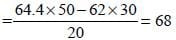SSC CGL Previous Year Questions: Average- 1 - Question 6

The average marks of 40 students was found to be 68. If the marks of two students were incorrectly entered as 48 and 64 instead of 84 and 46 respectively, then what is the correct average?       (SSC CGL-2018)

Detailed Solution for SSC CGL Previous Year Questions: Average- 1 - Question 6

Correct Average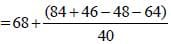= 68 + 0.45 = 68.45

SSC CGL Previous Year Questions: Average- 1 - Question 7

The difference between the average of first ten prime numbers and the first ten prime numbers of two digit is :        (SSC CHSL-2018)

Detailed Solution for SSC CGL Previous Year Questions: Average- 1 - Question 7

x = first ten prime numbers are, 2, 3, 5, 7, 11, 13, 17, 19, 23 and 29
y = first ten prime numbers of two digits are 11, 13, 17, 19, 23, 29 31, 37, 41 and 43.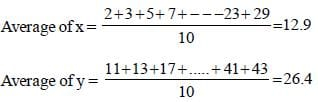Difference in Average = 26.4 – 12.9 = 13.5

SSC CGL Previous Year Questions: Average- 1 - Question 8

The average of 18 numbers is 52. The average of the first 8 numbers is 62 and the average of the next 7 numbers is 45.  If the 16th number is 6 less than the 17th number and the 17th number is one more than the 18th nmber, then what is the average of the 16th and 18th numbers?        (SSC Sub. Ins. 2018 )

Detailed Solution for SSC CGL Previous Year Questions: Average- 1 - Question 8

Sum of 18 numbers = 18 × 52 = 936
Sum of first 8 numbers = 62 × 8 = 496
Sum of next 7 numbers = 45 × 7 = 315
Sum of 16th, 17th and 18th numbers = 936 – 496 – 315= 125
Now, let 17th number is x.
then, 16th number is (x – 6).
and 18th number is (x – 1).
so, (x – 6) + x + (x – 1) = 125.
3x – 7 = 125 ⇒ 3x = 132.
x = 132/3 = 44.
Average of 16th and 18th number =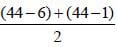= 40.5.

SSC CGL Previous Year Questions: Average- 1 - Question 9

In a match, average of runs scored by 5 players is 49. If the runs scored by four players are 75, 30, 62 and 21 respectively, then how many runs did the 5th player had scored?        (SSC Sub. Ins. 2017)

Detailed Solution for SSC CGL Previous Year Questions: Average- 1 - Question 9

Total runs scored by 5 players = (49 × 5) = 245
Total runs scored by 4 players = (75 + 30 + 62 + 21)
= 188
∴ Total runs did the 5th player had scored
= (245 – 188) = 57.

SSC CGL Previous Year Questions: Average- 1 - Question 10

What is the average of the squares of the first 19 natural numbers?       (SSC Sub. Ins. 2017)

Detailed Solution for SSC CGL Previous Year Questions: Average- 1 - Question 10

∵ Average of the square of the first 19 natural numbers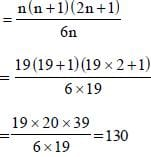SSC CGL Previous Year Questions: Average- 1 - Question 11

The average age of 30 students is 20 years and average age of 20 other students is 30 years. The average age of total number of students is:       (SSC MTS 2017)

Detailed Solution for SSC CGL Previous Year Questions: Average- 1 - Question 11

The average age of total number of students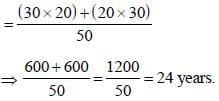SSC CGL Previous Year Questions: Average- 1 - Question 12

The mean of marks secured by 60 students in division A of class X is 64, 40 students of division B is 60 and that of 60 students of division C is 58. Find the mean of marks of the students of three divisions of Class X.         (SSC CHSL 2017)

Detailed Solution for SSC CGL Previous Year Questions: Average- 1 - Question 12

Mean of marks of the students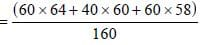= 9720/160 = 60.75

SSC CGL Previous Year Questions: Average- 1 - Question 13

The average runs conceded by a bowler in 5 matches is 45 and 15.75 in other 4 matches. What is the average runs conceded by the bowler in 9 matches?       (SSC CGL 2017)

Detailed Solution for SSC CGL Previous Year Questions: Average- 1 - Question 13

Required average runs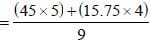= 288/9 = 32.

SSC CGL Previous Year Questions: Average- 1 - Question 14

The average age of 120 members of a society is 60.7 years. By addition of 30 new members, the average age becomes 56.3 years. What is the average age (in years) of newly joined members?        (SSC CGL 2017)

Detailed Solution for SSC CGL Previous Year Questions: Average- 1 - Question 14

Let average age of newly joined member = x
According to question,
120 × 60.7 + 30 × x = 150 × 56.3
7284 + 30x = 8445
∴ 30x = 8445 – 7284
30x = 1161
∴ x = 1161/30 = 38.7

SSC CGL Previous Year Questions: Average- 1 - Question 15

The average of 5 members of a family is 24 years. If the youngest member is 8 years old, then what was the average age (in years) of the family at the time of the birth of the youngest member?       (SSC CGL 2017)

Detailed Solution for SSC CGL Previous Year Questions: Average- 1 - Question 15

Required average age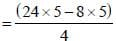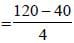= 80/4 = 20 years.

SSC CGL Previous Year Questions: Average- 1 - Question 16

The average of 17 results is 60. If the average of first 9 results is 57 and that of the last 9 results is 65, then what will be the value of 9th result?      (SSC CGL 2017)

Detailed Solution for SSC CGL Previous Year Questions: Average- 1 - Question 16

Value of 9th result = (9×57+9×65) – (17×60)
= 1098 – 1020
= 78

SSC CGL Previous Year Questions: Average- 1 - Question 17

The average monthly income of A and B is ₹15,050, the average monthly income of B and C is ₹15,350 and the average income of A and C is ₹15, 200. The monthly income of A is        (SSC Sub. Ins. 2016)

Detailed Solution for SSC CGL Previous Year Questions: Average- 1 - Question 17

Sum of monthly income of A + B = 30100 ...(i)
Sum of monthly income of B + C = 30700 ...(ii)
Sum of monthly income of A + C = 30400 ...(iii)
Subtracting eqn. (i) from (ii)
B + C – A – B = 30700 – 30100
C – A = 600 ...(iv)
C + A = 30400 ...(v)
Subtracting eqn. (v) from (iv)
C – A – C – A = 600 – 30400 – 2A = – 29800
A = ₹14900

SSC CGL Previous Year Questions: Average- 1 - Question 18

The average of 9 observations was found to be 35. Later on, it was detected that an observation 81 was misread as 18.The correct average of the observations is       (SSC CGL 2nd Sit. 2016)

Detailed Solution for SSC CGL Previous Year Questions: Average- 1 - Question 18

Average of 9 observations = 35
Total = 9 × 35 = 315
Misread Difference = 81 – 18 = 63
New total = 315 + 63 = 378
Average = 378/9 = 42
"Shortcut Method:
81 – 18 = 63
∴ 63/9 = 7
35 + 7 = 42"

SSC CGL Previous Year Questions: Average- 1 - Question 19

The average marks obtained by a class of 60 students is 65. The average marks of half of the students is found to be 85.The average marks of the remaining students is        (SSC CGL 2nd Sit. 2016)

Detailed Solution for SSC CGL Previous Year Questions: Average- 1 - Question 19

Total students = 60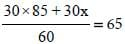30x = 65 × 60 – 30 × 85
30x = 30[65 × 2 – 85]
x = 30/30[130-85] = 45

SSC CGL Previous Year Questions: Average- 1 - Question 20

Visitors to a show were charged ₹ 15 each on the first day, ₹ 7.50 on the second day, ₹ 2.50 on the third day and total attendance on three days were in the ratio 2:5:13 respectively. The average charge per person for the entire three days is              (SSC CGL 2nd Sit. 2016)

Detailed Solution for SSC CGL Previous Year Questions: Average- 1 - Question 20

Let the attendance on the three day be 2x, 5x and 13x respecting.
Total charges = ₹(15× 2x + 7.50 × 5x + 2.50 × 13x )
= ₹(30x + 37.5x + 32.5x)
= ₹100x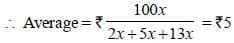## SSC CGL (Tier - 1) - Previous Year Papers (Topic Wise)

250 tests
Information about SSC CGL Previous Year Questions: Average- 1 Page
In this test you can find the Exam questions for SSC CGL Previous Year Questions: Average- 1 solved & explained in the simplest way possible. Besides giving Questions and answers for SSC CGL Previous Year Questions: Average- 1, EduRev gives you an ample number of Online tests for practice

250 tests